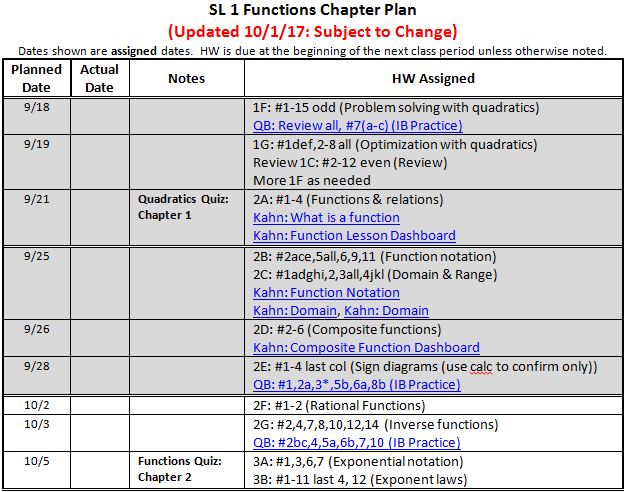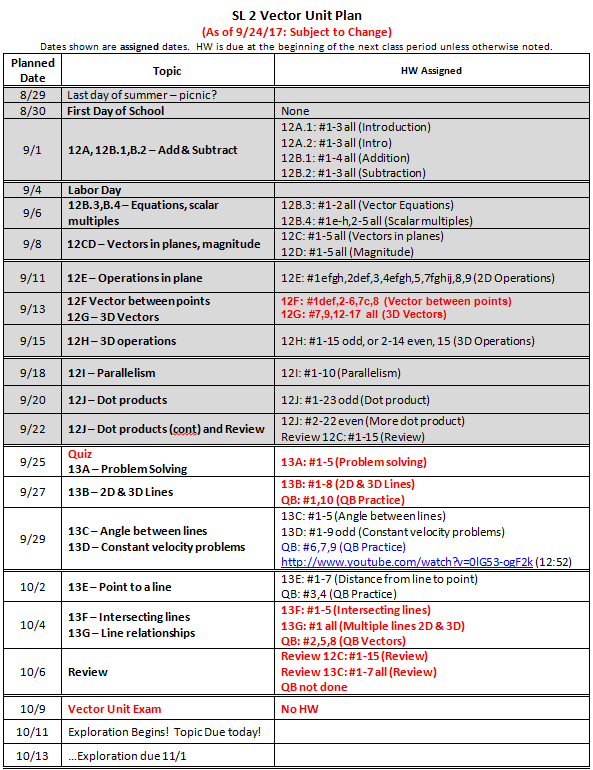### SL1 - 10/2/17

Two big ideas this week, and then our Functions Quiz on Thursday. We will move fast, so stay on top of things, ask lots of questions, and do your HW thoroughly...meaning, meaning, meaning. Lots of video support below - use it if you have questions! See you in class,
Mr. Alei

SL 1: Week of 10/2/17               (Previous Week - 9/25/17)

Mon 10/2:
2F: #1-2 (Rational Functions)
Kahn: Graphing Rational Functions
Tue 10/3:
2G: #2,4,7,8,10,12,14 (Inverse functions)
QB: #2bc,4,5a,6b,7,10(IB Practice)
Kahn: Inverse functions
Thu 10/5: Functions Quiz (Ch. 2)
3A: #1,3,6,7 (Exponential notation)
3B: #1-11 last 4, 12 (Exponent laws)

IB Questionbank, Class Notes, and other support
Functions Unit Plan
QB Quadratics Practice
QB Functions Practice
Smartboard Class Notes - Functions

Widgets to explore
Reciprocal and Rational Functions

Inverse Functions

Video support
Kahn Academy: What is a function
Kahn: Function Lesson DashboardThe fine print: See sidebar at right for links to previous assignments. Click on linked assignments to download notes for that section.  Dates above are when homework is assigned.  It is always due on the following class meeting. For access to electronic copies of the IB text books, see or email Mr. Alei.
Updated 9/24/17 8:51 am        ManageBac        SL Mind Map        IB      Desert

### SL2 - 10/2/17

Oops, I lost this post - rebuilt it vaguely....should have the correct assignments at least! See you in class,
Mr. Alei

SL 2: Week of 10/2/17               (Previous Week - 9/25/17)

Mon 10/2: Chapter 12 Test
13E: #1-7 (Distance from line to point)
QB: #3,4 (QB Practice)
Wed 10/4:
13F: #1-5 (Intersecting lines)
13G: #1 all (Multiple lines 2D & 3D) Too much, do a,c,e,g
QB: #2,5,8 (QB Vectors)
Fri 10/6:
Review 12C: #1-15 (Review)
Review 13C: #1-7 all (Review)
QB not done

Coming up:
Mon 10/9: Vector Unit Exam (Ch 12 & 13)

IB Questionbank, Class Notes, and other support
Vectors Unit Plan
QB Vector Practice
Smartboard Class Notes - Quadratics

Video support
Kahn Academy: Vector Index of TopicsThe fine print: See sidebar at right for links to previous assignments. Click on linked assignments to download notes for that section.  Dates above are when homework is assigned.  It is always due on the following class meeting. For access to electronic copies of the IB text books, see or email Mr. Alei.
Updated 9/24/17 2:02 pm        ManageBac        SL Mind Map        IB      Desert

### Jr. Seminar - 10/2/17

We'll finish up our sewing this week, either on Monday or Wednesday. Hopefully the weather will cooperate and we can also work with vehicles a little more. We'll see what else comes up :) See you in class,
Mr. Alei

Junior Seminar: Week of 10/2/17               (Previous Week - 9/25/17)

Mon 10/2:
Mindfulness - body scan?
Discussion of Glow kids
Wed 10/4:
Finish sewing projects
Tie some knots
Fri 10/6:
Plan was for Jump starting & Changing a tire
But we ended up helping Augie build a wall

Resources
Mindfulness
Mindful.org web site
Harvard SPH: Benefits of Mindfulness Practice
Psych Today: 10 Benefits of Mindfulness
Psych Today: Possible Pitfalls of Mindfulness

Technology
Glow Kids: Yahoo! Interview (13:58)
Glow Kids: Full Presentation (1:47:33)

### BYU Calc 2 - 10/2/17

It looks like we're off and running smoothly. Be sure to be present on Mondays and stay up with your schedule, which is available here. I will expect you to be up on this unless you hear otherwise from me. Be in touch,
Mr. Alei

BYU Calc 2: Last Updated 10/2/2017              (Previous Post - 9/4/17)

Some helpful links
BYU Course Policies
Our shared timeline

Kahn Academy Video links
Limits
Continuity
Differentiability
L'Hospital's Rule
Extreme Value Theorem
Intermediate Value Theorem
Mean Value Theorem (Rolle's)
Hyperbolic functions
Riemann Sums

Prerequisites
Successful completion of MATH 113 requires a basic knowledge of the concepts of MATH 112 (Calculus 1). These topics are not reviewed in the course. Students are expected to have competency in these areas before starting MATH 113. Here are the skills that are important for students to already know how to do:
1. Limits
1. Explain intuitively and graphically the concept of the limit of a function.
2. Recognize the correct definition of a limit and be able to use the definition of a limit to prove simple limit statements.
3. Recall and use limit theorems to evaluate limits.
4. Explain and use one-sided limits, limits at infinity, and infinite limits.
5. Apply limits to the description of the asymptotes of a function.
6. Find lim x → a f ( x ) for functions which are not defined at a.
2. Continuity
1. Recognize the definition of continuity at a point.
2. Explain the graphical interpretation of continuity.
3. Understand different types of discontinuities and which can be rewritten so as to be continuous.
4. Use continuity in evaluating limits of composite functions.
5. Apply the Extreme Value and Intermediate Value theorems.
6. State these two theorems correctly.
3. Derivatives
1. Explain and apply the graphical interpretation of a derivative as slope.
2. Explain and apply the dynamic interpretation of the derivative as the rate of change.
3. Define a derivative and compute the derivative of a function.
4. Use the differentiation formulas to find the derivative of any elementary function (polynomial, rational, root, exponential, logarithmic, trigonometric, inverse trigonometric, and hyperbolic functions, as well as all combinations and compositions thereof).
5. Recognize and use the common notations for a derivative.
6. Recall and use the relationship between differentiability and continuity.
7. Use implicit differentiation to find the first derivative of an implicitly defined function.
8. Explain and use the interpretations of the second derivative.
9. Compute derivatives of a higher order.
10. Be proficient in all the differentiation techniques, including the product rule and chain rule.
4. Rolle’s theorem and the mean value theorem
1. Recall and explain the meaning of Rolle’s theorem and the mean value theorem.
2. Use the derivative to describe the monotonicity of a function.
3. Use the second derivative to describe the concavity of a function.
4. Use the first and second derivative tests to classify extrema.
5. Use the derivatives to find critical points, inflection points, and local extrema.
6. Use derivatives to aid in sketching by hand the graph of a function.
7. Solve optimization problems.
8. Solve related rates problems.
9. Use l’Hôpital’s rule to evaluate limits.
5. Definite integrals
1. Explain and apply the graphical interpretation of the definite integral as area.
2. Explain and apply the dynamic interpretation of the definite integral as total change (given the velocity or acceleration, find the displacement.)
3. Recognize a correct definition of the definite integral.
4. Recall and use the definition of the definite integral as a limit of Riemann sums (that is, find what a certain limit of Riemann sums is in terms of an integral).
5. Recognize an integral that corresponds to a sequence of Riemann sums.
6. Recall and use linearity and interval properties of definite integrals.
7. Explain that interval properties are properties pertaining to the interval of integration like ∫ a b f ( x ) ⅆ x = - ∫ b a f ( x ) ⅆ x and ∫ a b f ( x ) ⅆ x + ∫ b c f ( x ) ⅆ x = ∫ a c f ( x ) ⅆ x .
8. Recall and explain the Fundamental Theorem of Calculus.
9. Find derivatives of functions defined as definite integrals with variable limits, including situations which will require the use of other rules of differentiation in conjunction with the Fundamental Theorem of Calculus.
10. Use the Fundamental Theorem to evaluate definite integrals by antidifferentiation.
11. Use a simple substitution to find an antiderivative.
NOTE: In order to assess readiness and before students can get access to the homework through WebAssign, each student is required to take a pretest that covers the above material. This pretest is free. Each student is allowed only 2 attempts and the pretest will count towards the student’s course grade.
The fine print: See sidebar at right for links to previous assignments. Click on linked assignments to download notes for that section.  Dates above are when homework is assigned.  It is always due on the following class meeting.
Updated 5/14/17 1:09 pm        ManageBac B Block        ManageBac A Block        SL Mind Map